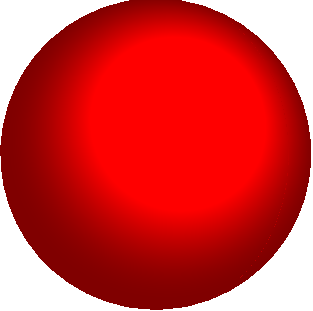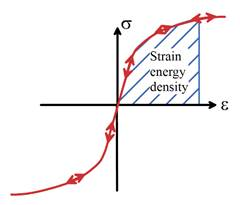3.3 Hypoelasticity $–$ elastic materials with a nonlinear stress-strain relation under small deformationHypoelasticity is used to model materials that exhibit nonlinear, but reversible, stress strain behavior even at small strains.  Its most common application is in the so-called `deformation theory of plasticity,’ which is a crude approximation of the behavior of metals loaded beyond the elastic limit.

A hypoelastic material has the following propertiesThe solid has a preferred shapeThe specimen deforms reversibly:  if you remove the loads, the solid returns to its original shape.The strain in the specimen depends only on the stress applied to it $–$ it doesn’t depend on the rate of loading, or the history of loading.The stress is a nonlinear function of strain, even when the strains are small, as shown in the picture above.  Because the strains are small, this is true whatever stress measure we adopt (Cauchy stress or nominal stress), and is true whatever strain measure we adopt (Lagrange strain or infinitesimal strain).We will assume here that the material is isotropic (i.e. the response of a material is independent of its orientation with respect to the loading direction).  In principle, it would be possible to develop anisotropic hypoelastic models, but this is rarely done.

The stress strain law is constructed as follows:Strains and rotations are assumed to be small.  Consequently, deformation is characterized using the infinitesimal strain tensor ${\epsilon }_{ij}$ defined in Section 2.1.7.   In addition, all stress measures are taken to be approximately equal.  We can use the Cauchy stress ${\sigma }_{ij}$ as the stress measure.

###When we develop constitutive equations for nonlinear elastic materials, it is usually best to find an equation for the strain energy density of the material as a function of the strain, instead of trying to write down stress-strain laws directly.  This has several advantages: (i) we can work with a scalar function; and (ii) the existence of a strain energy density guarantees that deformations of the material are perfectly reversible.If the material is isotropic, the strain energy density can only be a function strain measures that do not depend on the direction of loading with respect to the material.   One can show that this means that the strain energy can only be a function of invariants of the strain tensor $–$ that is to say, combinations of strain components that have the same value in any basis (see Appendix B).  The strain tensor always has three independent invariants: these could be the three principal strains, for example.   In practice it is usually more convenient to use the three fundamental scalar invariants:

${I}_{1}={\epsilon }_{kk}\text{\hspace{0.17em}}\text{\hspace{0.17em}}\text{\hspace{0.17em}}\text{\hspace{0.17em}}\text{\hspace{0.17em}}\text{\hspace{0.17em}}\text{\hspace{0.17em}}\text{\hspace{0.17em}}\text{\hspace{0.17em}}\text{\hspace{0.17em}}\text{\hspace{0.17em}}{I}_{2}=\frac{1}{2}\left({\epsilon }_{ij}{\epsilon }_{ij}-{\epsilon }_{kk}{\epsilon }_{pp}/3\right)\text{\hspace{0.17em}}\text{\hspace{0.17em}}\text{\hspace{0.17em}}\text{\hspace{0.17em}}\text{\hspace{0.17em}}\text{\hspace{0.17em}}\text{\hspace{0.17em}}\text{\hspace{0.17em}}\text{\hspace{0.17em}}{I}_{3}=\mathrm{det}\left(\epsilon \right)=\frac{1}{6}{\in }_{ijk}{\in }_{lmn}{\epsilon }_{li}{\epsilon }_{mj}{\epsilon }_{nk}$

### Here, ${I}_{1}$ is a measure of the volume change associated with the strain; ${I}_{2}$ is a measure of the shearing caused by the strain, and I can’t think of a good physical interpretation for ${I}_{3}$.  Fortunately, it doesn’t often appear in constitutive equations.

Strain energy density:

In principle, the strain energy density could be any sensible function $U\left({I}_{1},{I}_{2},{I}_{3}\right)$.   In most practical applications, nonlinear behavior is only observed when the material is subjected to shear deformation (characterized by ${I}_{2}$ ); while stress varies linearly with volume changes (characterized by ${I}_{1}$ ).   This behavior can be characterized by a strain energy density

$U=\frac{1}{6}K{I}_{1}^{2}+\frac{2n{\sigma }_{0}{\epsilon }_{0}}{n+1}{\left(\frac{{I}_{2}}{{\epsilon }_{0}^{2}}\right)}^{\left(n+1\right)/2n}$

where $K,{\sigma }_{0},{\epsilon }_{0},n$ are material properties (see below for a physical interpretation).

Stress-strain behavior

For this strain energy density function, the stress follows as

${\sigma }_{ij}=\frac{\partial U}{\partial {\epsilon }_{ij}}=\frac{K}{3}{\epsilon }_{kk}{\delta }_{ij}+{\sigma }_{0}{\left(\frac{{I}_{2}}{{\epsilon }_{0}^{2}}\right)}^{\left(1-n\right)/2n}\left(\frac{{\epsilon }_{ij}-{\epsilon }_{kk}{\delta }_{ij}/3}{{\epsilon }_{0}}\right)$

The strain can also be calculated in terms of stress

${\epsilon }_{ij}=\frac{1}{3K}{\sigma }_{kk}{\delta }_{ij}+{\epsilon }_{0}{\left(\frac{{J}_{2}}{{\sigma }_{0}^{2}}\right)}^{\left(n-1\right)/2}\left(\frac{{\sigma }_{ij}-{\sigma }_{kk}{\delta }_{ij}/3}{{\sigma }_{0}}\right)$

where ${J}_{2}=\left({\sigma }_{ij}{\sigma }_{ij}-{\sigma }_{kk}{\sigma }_{pp}/3\right)/2$ is the second invariant of the stress tensor.

To interpret these results, note that

###If the solid is subjected to uniaxial tension, (with stress ${\sigma }_{11}=\sigma$ and all other stress components zero); the nonzero strain components are

${\epsilon }_{11}=\frac{\sigma }{3K}+\frac{2}{\sqrt{3}}{\epsilon }_{0}{\left(\frac{\sigma }{\sqrt{3}{\sigma }_{0}}\right)}^{n}\text{\hspace{0.17em}}\text{\hspace{0.17em}}\text{\hspace{0.17em}}\text{\hspace{0.17em}}\text{\hspace{0.17em}}\text{\hspace{0.17em}}\text{\hspace{0.17em}}{\epsilon }_{22}={\epsilon }_{33}=\frac{\sigma }{3K}-\frac{1}{\sqrt{3}}{\epsilon }_{0}{\left(\frac{\sigma }{\sqrt{3}{\sigma }_{0}}\right)}^{n}$

###If the solid is subjected to hydrostatic stress (with ${\sigma }_{11}={\sigma }_{22}={\sigma }_{33}=\sigma$ and all other stress components zero) the nonzero strain components are

${\epsilon }_{11}={\epsilon }_{22}={\epsilon }_{33}=\frac{\sigma }{K}$

###If the solid is subjected to pure shear stress (with ${\sigma }_{12}={\sigma }_{21}=\tau$ and all other stress components zero) the nonzero strains are

${\epsilon }_{12}={\epsilon }_{21}={\epsilon }_{0}{\left(\frac{\tau }{{\sigma }_{0}}\right)}^{n}$

Thus, the solid responds linearly to pressure loading, with a bulk modulus K.  The relationship between shear stress and shear strain is a power law, with exponent n

This is just an example of a hypoelastic stress-strain law $–$ many other forms could be used.